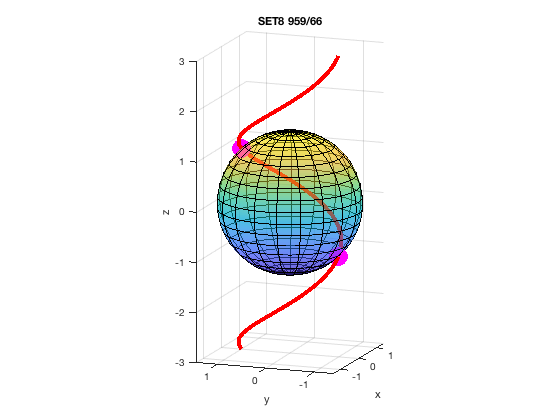# MATH 251: Calculus 3, SET8

## 14: Partial Derivatives

### 14.6: Directional Derivatives and the Gradient Vector

These problems are done with the CAS. See Hand Solutions for details.

#### 1. [956/4]

A directional derivative is computed. The unit direction vector is given by u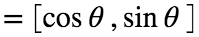.
syms x y
P = sym([1 2]), u = sym([cos(pi/3) sin(pi/3)])
P =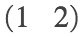u =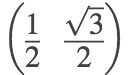f =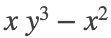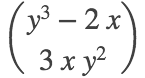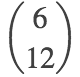dir_deriv =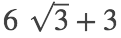dir_deriv_appx = double(dir_deriv)
dir_deriv_appx = 13.3923

#### 2. [957/10]

Similar to #1 but with a 3-variable function.
syms x y z
P = sym([0 1 -1]), u = sym([3 4 12]/13)
P =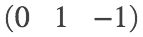u =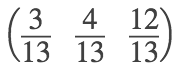f =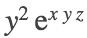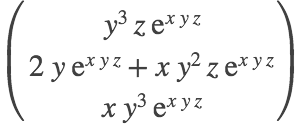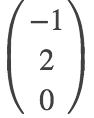dir_deriv =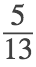dir_deriv_appx = double(dir_deriv)
dir_deriv_appx = 0.3846

#### 3. [957/20]

Here the unit direction vector is the vector from P to Q divided by its length.
syms x y z
P = sym([2 1 1]), Q = sym([0 -3 5]), PQ = Q-P, u = PQ/norm(PQ)
P =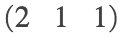Q =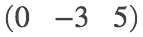PQ =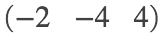u =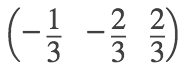f =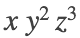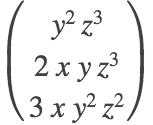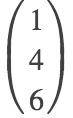dir_deriv = 1

#### 4. [957/26]

The direction of the maximum rate of change is that of the gradient vector at the point.
The maximum rate of change itself is the magnitude of said vector.
syms p q r real
P = sym([1 2 1]), f = atan(p*q*r), grad_f = gradient(f, [p q r])
P =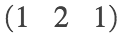f =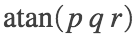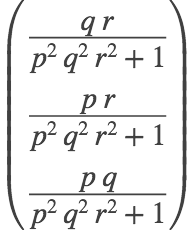grad_f_P = subs(grad_f, [p q r], P)' % direction of maximum rate of change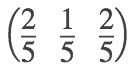u = grad_f_P / norm(grad_f_P) % (or use unit vector thereof, but extra work!)
u =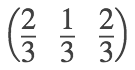max_rate_of_change =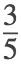#### 5. [957/30]

As the fisherman heads toward the buoy, the water gets deeper. (Its depth is decreasing.)
syms x y
P = sym([80 60]), Q = sym([0 0]), PQ = Q-P, u = PQ/norm(PQ)
P =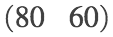Q =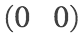PQ =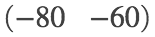u =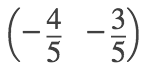z = 200 + 0.02*x^2 - 0.001*y^3, grad_z = gradient(z, [x y])
z =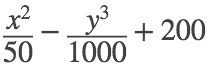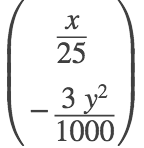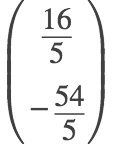dir_deriv =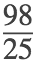dir_deriv_appx = double(dir_deriv) % in meters per second
dir_deriv_appx = 3.9200

#### 6. [958/46]

syms t u x y z
% Let F = LHS - RHS for the surface equation given, understood to be set 0.
F = x^4 + y^4 + z^4 - 3 * x^2 * y^2 * z^2, P = sym([1,1,1])
F =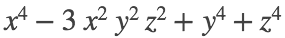P =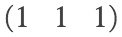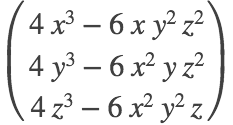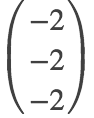n = sym([1 1 1]) % parallel to grad_F_P (cleaner): the normal vector to surface at P
n =tangent_plane = dot(n,[x y z]) == dot(n,P)
tangent_plane =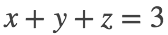normal_line = P + t*n
normal_line =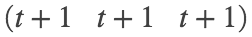normal_line_reparameterized = sym([u u u])
normal_line_reparameterized =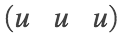#### 7. [959/54]

The point on an ellipsoid at which the tangent plane is parallel to a given plane is found.
syms c x y z real
% Let F = LHS - RHS for the surface equation given, understood to be set 0.
F = x^2 + y^2 + 2*z^2 - 1, ellipsoid = F == 0
F =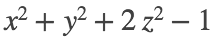ellipsoid =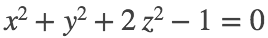grad_F =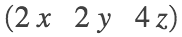n = 1×3
1 2 1
eq1 = grad_F == c*n % Normal to surface must be parallel to normal to given plane.
eq1 =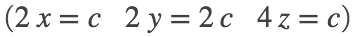eq2 = solve(eq1, [x y z]); v = [eq2.x eq2.y eq2.z]
v =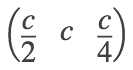eq3 = subs(ellipsoid, [x y z], v)
eq3 =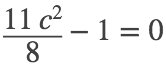s = solve(eq3, c) % Two multipliers found.
s =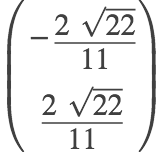P1 = subs(v, c, s(1)), P1_appx = double(P1) % first point
P1 =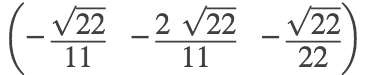P1_appx = 1×3
-0.4264 -0.8528 -0.2132
P2 = subs(v, c, s(2)), P2_appx = double(P2) % second point
P2 =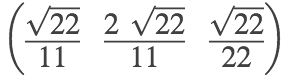P2_appx = 1×3
0.4264 0.8528 0.2132

#### 8. [959/60]

Points are found at which the normal line to an ellipsoid at a given point intersects a sphere.
syms t x y z real; P = sym([1 2 1])
P =% Let F = LHS - RHS for the surface equation given, understood to be set 0.
F = 4*x^2 + y^2 + 4*z^2 - 12, ellipsoid = F == 0, S = x^2 + y^2 + z^2 == 102 % S: sphere
F =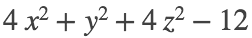ellipsoid =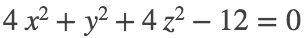S =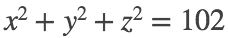grad_F =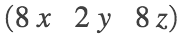n =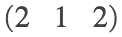NL = P + n*t, eq = subs(S, [x y z], NL) % Normal line intersects sphere.
NL =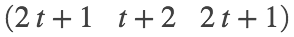eq =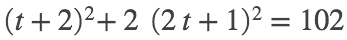soln = solve(eq, t)
soln =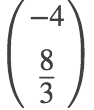P1 = subs(NL, t, soln(1)), P2 = subs(NL, t, soln(2)) % points of intersection
P1 =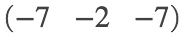P2 =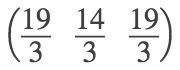#### 9. [959/64]

A plane and circular cylinder intersect in an ellipse, a conic section. Parametric equations for the tangent line to this ellipse at a specific point are found.
syms t u x y z phi theta
plane = y + z == 3, cylin = x^2 + y^2 == 5, s5 = sym(sqrt(5))
plane =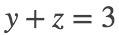cylin =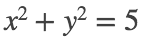s5 =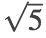r(t) = [s5*cos(t) s5*sin(t) 3 - s5*sin(t)] % parameterized ellipse of intersection
r(t) =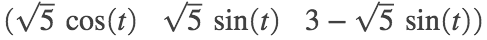ti = acos(sym(s5/5)), P = r(ti) % designated point and corresponding parameter value
ti =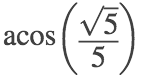P =Dr = diff(r,t), v = Dr(ti)
Dr(t) =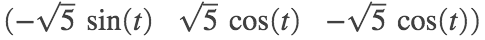v =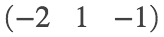L(t) = P + u*v % tangent line to ellipse at point
L(t) =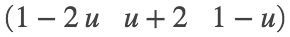#### 10. [959/66]

Similar to #8, but with a helix instead of a normal line. An illustration is provided.
syms t x y z
helix = [cos(pi*t/2), sin(pi*t/2), t], S = x^2 + y^2 + z^2 == 2 % sphere
helix =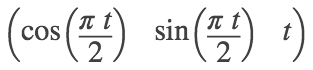S =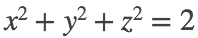eq1 = subs(S, [x y z], helix) % Simplified equation for intersection gives t values.
eq1 =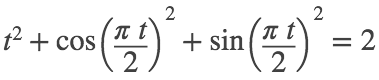soln = solve(eq1, t), P1 = subs(helix, t, 1), P2 = subs(helix, t, -1) % pts of intersection
soln =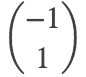P1 =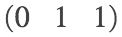P2 =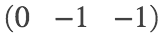n1 = P1, n2 = P2 % For a sphere centered at the origin, position vectors are normal vectors.
n1 =n2 =Dr(t) = diff(helix, t), v1 = Dr(1), v2 = Dr(-1)
Dr(t) =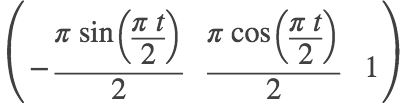v1 =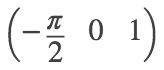v2 =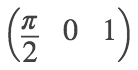ang1 = acos(dot(n1,v1) / (norm(n1)*norm(v1))), ang1_appx = rad2deg(double(ang1)) % degrees
ang1 =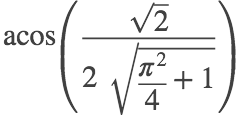ang1_appx = 67.6826
ang2 = acos(dot(n2,v2) / (norm(n2)*norm(v2))), ang2_appx = rad2deg(double(ang2)) % degrees
ang2 =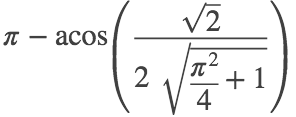ang2_appx = 112.3174
%
syms phi theta; s2 = sym(sqrt(2))
s2 =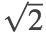xs = s2*sin(phi)*cos(theta), ys = s2*sin(phi)*sin(theta), zs = s2*cos(phi)
xs =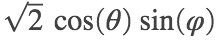ys =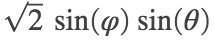zs =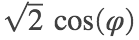figure
fsurf(xs, ys, zs, [0 pi 0 2*pi], 'MeshDensity', 20); hold on
%
xt = helix(1), yt = helix(2), zt = helix(3)
xt =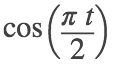yt =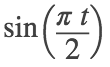zt = t
fplot3(xt, yt, zt, [-3 3], 'r', 'LineWidth', 4)
%
plot3(P1(1), P1(2), P1(3), 'mo', 'MarkerFaceColor', 'm', 'MarkerSize', 18)
plot3(P2(1), P2(2), P2(3), 'mo', 'MarkerFaceColor', 'm', 'MarkerSize', 18)
%
axis equal; xticks(-1:1); yticks(-1:1); zticks(-3:3)
xlabel('x'); ylabel('y'); zlabel('z')
title('SET8 959/66'); alpha 0.5
view(-70,13)